# NCERT Solutions For Class 10 Maths Chapter 15 Probability

NCERT Solutions For Class 10 Maths Chapter 15 Probability

## NCERT Solutions For Class 10 Maths Chapter 15 Probability Ex 15.1

Ex 15.1 Class 10 Maths Question 1.
Complete the following statements:
(i) Probability of an event E + Probability of the event ‘not E’ = ………
(ii) The probability of an event that cannot happen is ……… Such an event is called ………
(iii) The probability of an event that is certain to happen is ………. Such an event is called ………
(iv) The sum of the probabilities of all the elementary events of an experiment is ………..
(v) The probability of an event is greater than or equal to …………. and less than or equal to ………..
Solution:You can also download the free PDF of  Ex 15.1 Class 10 Probability NCERT Solutions or save the solution images and take the print out to keep it handy for your exam preparation.

Ex 15.1 Class 10 Maths Question 2.
Which of the following experiments have equally likely outcomes? Explain.
(i) A driver attempts to start a car. The car starts or does not start.
(ii) A player attempts to shoot a basketball. She/he shoots or misses the shot.
(iv) A baby is born. It is a boy or a girl.
Solution: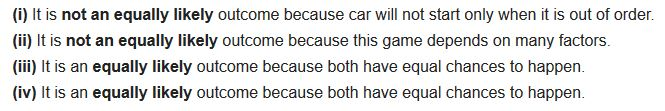Ex 15.1 Class 10 Maths Question 3.
Why is tossing a coin considered to be a fair way of deciding which team should get the bail at the beginning of a football game?
Solution:Ex 15.1 Class 10 Maths Question 4.
Which of the following cannot be the probability of an event?
(A) 23
(B) -1.5
(C) 15%
(D) 0.7
Solution: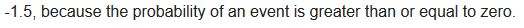Ex 15.1 Class 10 Maths Question 5.
If P (E) = 0.05, what is the probability of ‘not E’?
Solution:Ex 15.1 Class 10 Maths Question 6.
A bag contains lemon flavoured candies only. Malini takes out one candy without looking into the bag. What is the probability that she takes out
(i) an orange flavoured candy?
(ii) a lemon flavoured candy?
Solution:Ex 15.1 Class 10 Maths Question 7.
It is given that in a group of 3 students, the probability of 2 students not having the same birthday is 0.992. What is the probability that the 2 students have the same birthday?
Solution: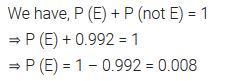Ex 15.1 Class 10 Maths Question 8.
A bag contains 3 red balls and 5 black balls. A ball is drawn at random from the bag. What is the probability that the ball drawn is
(i) red?
(ii) not red?
Solution: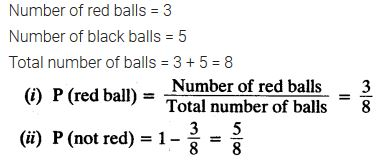Ex 15.1 Class 10 Maths Question 9.
A box contains 5 red marbles, 8 white marbles and 4 green marbles. One marble is taken out of the box at random. What is the probability that the marble taken out will be
(i) red?
(ii) white?
(iii) not green?
Solution:Ex 15.1 Class 10 Maths Question 10.
A piggy bank contains hundred 50 p coins, fifty ₹ 1 coins, twenty ₹ 2 coins and ten ₹ 5 coins. If it is equally likely that one of the coins will fall out when the bank is turned upside down, what is the probability that the coin
(i) will be a 50 p coin?
(ii) will not be a ₹ 5 coin?
Solution: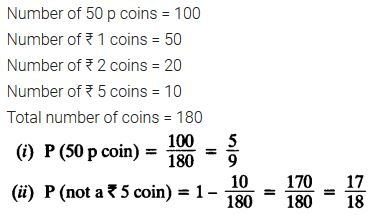Ex 15.1 Class 10 Maths Question 11.
Gopi buys a fish from a shop for his aquarium. The shopkeeper takes out one fish at random from a tank containing 5 male fish and 8 female fish (see figure). What is the probability that the fish taken out is a male fish?Solution: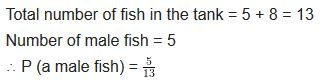Ex 15.1 Class 10 Maths Question 12.
A game of chance consists of spinning an arrow which comes to rest pointing at one of the numbers 1, 2, 3, 4, 5, 6, 7, 8 (see figure.), and these are equally likely outcomes. What is the probability that it will point at
(i) 8?
(ii) an odd number?
(iii) a number greater than 2?
(iv) a number less than 9?Solution:Ex 15.1 Class 10 Maths Question 13.
A die is thrown once. Find the probability of getting
(i) a prime number
(ii) a number lying between 2 and 6
(ill) an odd number
Solution:Ex 15.1 Class 10 Maths Question 14.
One card is drawn from a well-shuffled deck of 52 cards. Find the probability of getting
(i) a king of red colour
(ii) a face card
(iii) a red face card
(iv) the jack of hearts
(vi) the queen of diamonds
Solution: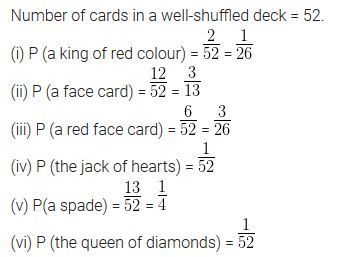Ex 15.1 Class 10 Maths Question 15.
Five cards – the ten, jack, queen, king and ace of diamonds, are well shuffled with their face downwards. One card is then picked up at random.
(i) What is the probability that the card is the queen?
(ii) If the queen is drawn and put aside, what is the probability that the second card picked up is
(a) an ace?
(b) a queen?
Solution:Ex 15.1 Class 10 Maths Question 16.
12 defective pens are accidentally mixed with 132 good ones. It is not possible to just look at a pen and tell whether or not it is defective. One pen is taken out at random from this lot. Determine the probability that the pen taken out is a good one.
Solution: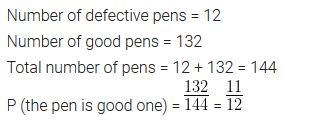Ex 15.1 Class 10 Maths Question 17.
(i) A lot of 20 bulbs contain 4 defective ones. One bulb is drawn at random from the lot. What is the probability that this bulb is defective?
(ii) Suppose the bulb drawn in (i) is not defective and is not replaced. Now one bulb is drawn at random from the rest. What is the probability that this bulb is not defective?
Solution:Ex 15.1 Class 10 Maths Question 18.
A box contains 90 discs which are numbered from 1 to 90. If one disc is drawn at random from the box, find the probability that it bears
(i) a two digit number.
(ii) a perfect square number.
(iii) a number divisible by 5.
Solution:Ex 15.1 Class 10 Maths Question 19.
A child has a die whose six faces show the letters as given below:The die is thrown once. What is the probability of getting
(i) A?
(ii) D?
Solution: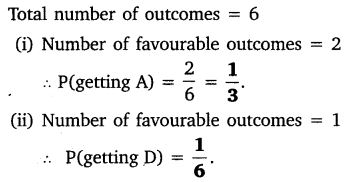Ex 15.1 Class 10 Maths Question 20.
Suppose you drop a die at random on the rectangular region shown in figure. What is the probability that it will land inside the circle with diameter 1 m?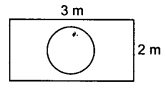Solution: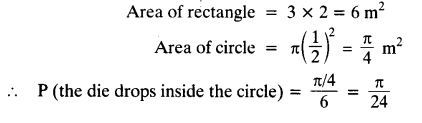Ex 15.1 Class 10 Maths Question 21.
A lot consists of 144 ball pens of which 20 are defective and the others are good. Nuri will buy a pen if it is good, but will not buy if it is defective. The shopkeeper draws one pen at random and gives it to her. What is the probability that
(ii) she will not buy it?
Solution:Ex 15.1 Class 10 Maths Question 22.
Two dice, one blue and one grey, are thrown at the same time. Now
(i) Complete the following table:(ii) A student argues that-there are 11 possible outcomes 2, 3, 4, 5, 6, 7, 8, 9, 10, 11 and 12. Therefore, each of them has a probability 1/11. Do you agree with this argument? Justify your answer.
Solution:Ex 15.1 Class 10 Maths Question 23.
A game consists of tossing a one rupee coin 3 times and noting its outcome each time. Hanif wins if all the tosses give the same result, i.e. three heads or three tails, and loses otherwise. Calculate the probability that Hanif will lose the game.
Solution:Ex 15.1 Class 10 Maths Question 24.
A die is thrown twice. What is the probability that
(i) 5 will not come up either time?
(ii) 5 will come up at least once?
[Hint: Throwing a die twice and throwing two dice simultaneously are treated as the same experiment.]
Solution: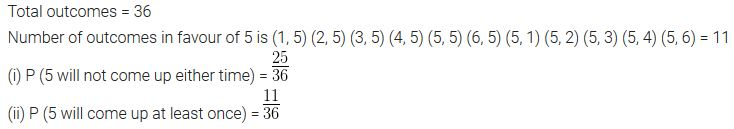Ex 15.1 Class 10 Maths Question 25.
Which of the following arguments are correct and which are not correct? Give reasons for your answer.
(i) If two coins are tossed simultaneously there are three possible outcomes- two heads, two tails or one of each. Therefore, for each of these outcomes, the probability is 1/3.
(ii) If a die is thrown, there are two possible outcomes- an odd number or an even number. Therefore, the probability of getting an odd number is 1/2.
Solution:### Class 10 Maths Probability Mind Maps

#### Probability – An Experimental (Empirical) Approach

Let n be the total number of trails. The empirical probability of an event E happening, is given by(i) Experiment : An operation which can produce some well defined outcomes is known as experiment.
(ii) Trail : Performing of an experiment is called trial.
(iii) Equally likely outcomes : Outcomes of trial are equally likely if there is no reason to accept one in preference to the others.
(iv) Sample space : The set of all possible outcomes of an experiment is called sample space.
(v) Elementary event : An event having only one outcome
Note that the sum of probabilities of all the elementary events of an experiment is 1.

#### Probability – A Theoretical Approach(Classical Probability)

If an event ‘A’ can happen is ‘m’ ways and does not happen in ‘n’ ways, then the probability of occurrence of event ‘A’ denoted by P(A) is given by
Number of favourable outcomes m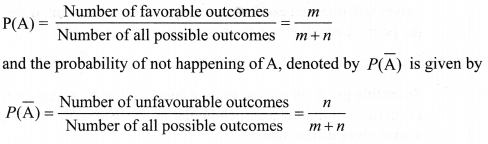Probability of Impossible and Sure Events
The probability of an event which is impossible to occur is 0 and such an event is called impossible event, i.e; for impossible event T, P(I) = 0
The probability of an event which is sure or certain to occur is 1 and such an event is called sure event or certain event.
i.e; for sure event or certain event ‘s’, P(s) = 1

#### Range of the Probability of an Event

From the definition of the probability P(E), we see that the numerator (number of outcomes favourable to the event E) is always equal or greater than 0 but less than or equal to the denominator (the number of all possible outcomes). Therefore,
0 ≤ P(E) ≤ 1

#### Complementary Events

The event representing (‘not E) is called the complement of event ‘E’ and we say that the events E and E¯¯¯¯ are complementary events,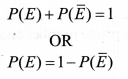## NCERT Solutions For Class 10 Maths Chapter 15 Probability Ex 15.2

Ex 15.2 Class 10 Maths Question 1.
Two customers Shyam and Ekta are visiting a particular shop in the same week (Tuesday to Saturday). Each is equally likely to visit the shop on any day as on another day. What is the probability that both will visit the shop on
(i) the same day?
(ii) consecutive days?
(iii) different days?
Solution: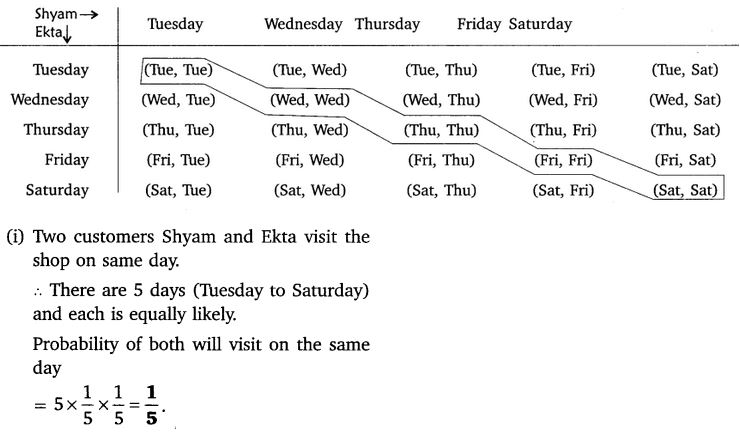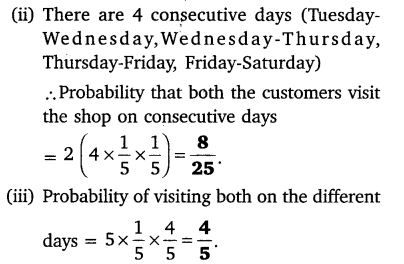Ex 15.2 Class 10 Maths Question 2.
A die is numbered in such a way that its faces show the number 1, 2, 2, 3, 3, 6. It is thrown two times and the total score in two throws is noted. Complete the following table which gives a few values of the total score on the two throws:What is the probability that the total score is at least 6?
(i) even
(ii) 6
(iii) at least 6
Solution:Ex 15.2 Class 10 Maths Question 3.
A bag contains 5 red balls and some blue balls. If the probability of drawing a blue ball is doubles that of a red ball, determine the number of blue balls in the bag.
Solution: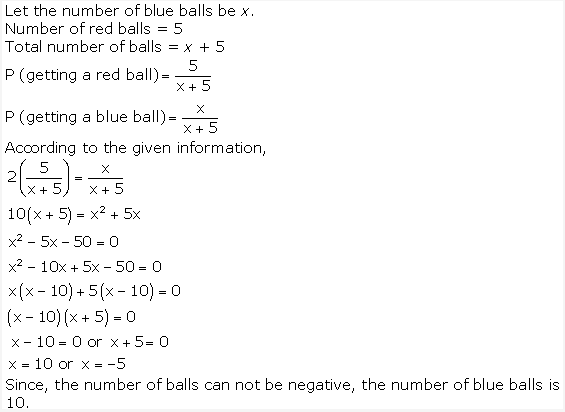Ex 15.2 Class 10 Maths Question 4.
A box contains 12 balls out of which x are black. If one ball is drawn at random from the box, what is the probability that it will be a black ball? If 6 more black balls are put in the box, the probability of drawing a black ball is now double of what it was before. Find x.
Solution:Ex 15.2 Class 10 Maths Question 5.
A jar contains 24 marbles, some are green and others are blue. If a marble is drawn at random from the jar, the probability that it is green is 2/3. Find the number of blue balls in the jar.
Solution:## Probability Class 10 Extra Questions Maths Chapter 15## Important Questions for Class 10 Maths Chapter 15 Probability

### Probability Class 10 Important Questions Very Short Answer (1 Mark)

Question 1.
In a family of 3 children calculate the probability of having at least one boy. (2014OD)
Solution:
S = {bbb, bbg, ggb, ggg}
Atleast 1 boy = {bbb, bbg, ggb}
∴ P(atleast 1 boy) = 3/4

Question 2.
A letter of English alphabet is chosen at random. Determine the probability that the chosen letter is a consonant. (2015D)
Solution:
Total English alphabets = 26
Number of consonants = 21
∴ P (letter is a consonant) = 21/26

Question 3.
A card is drawn at random from a well shuffled pack of 52 playing cards. Find the probability of getting neither a red card nor a queen. (2016OD)
Solution:
S = 52
P(neither a red card nor a queen)
= 1 – P(red card or a queen)Question 4.
A box contains cards numbered 6 to 50. A card is drawn at random from the box. Calculate the probability that the drawn card has a number which is a perfect square. (2013OD)
Solution:
Total number of cards = 50 – 6 + 1 = 45
Perfect square numbers are 9, 16, 25, 36, 49, i.e.,
5 numbers
∴ P(a prefect square) = 5/45=1/9

Question 5.
Cards marked with number 3, 4, 5, …, 50 are placed in a box and mixed thoroughly. A card is drawn at random from the box. Find the probability that the selected card bears a perfect square number. (2016D)
Solution:
Total no. of cards = 50 – 3 + 1 = 48
Perfect square number cards are 4, 9, 16, 25, 36, 49 i.e., 6 cards
∴ P(perfect square number) = 6/48=1/8

Question 6.
A number is chosen at random from the numbers -3, -2, -1, 0, 1, 2, 3. What will be the probability that square of this number is less than or equal to 1? (2017D)
Solution:
(-3)2 = 9; (-2)2 = 4; (-1)2 = 1; (0)2 = 0
(1)2 = 1; (2)2 = 4; (3)22 = 9
∴ P(Sq. of nos. of ≤ 1) = 3/7

Question 7.
If two different dice are rolled together, calculate the probability of getting an even number on both dice. (2014D)
Solution:
Two dice can be thrown as 6 × 6 = 36 ways
Even numbers on both the dice can be obtained as∴ P(even numbers) = 9/36=1/4

Question 8.
Two different dices are tossed together. Find the probability that the product of the two numbers on the top of the dice is 6. (2015OD)
Solution:
Total outcomes = 6= 62 = 36
Possible outcomes having the product of the two numbers on the top of the dice as 6 are
(3 × 2, 2 × 3, 6 × 1, 1 × 6), i.e, 4
P(Product of two nos. is 6) = 4/36=1/9

Question 9.
The probability of selecting a rotten apple ran domly from a heap of 900 apples is 0.18. What is the number of rotten apples in the heap? (2017OD)
Solution:∴ No. of rotten apples = 900 × 0.18 = 162

### Probability Class 10 Important Questions Short Answer-I (2 Marks)

Question 10.
A coin is tossed two times. Find the probability of getting both heads or both tails. (2011D)
Solution:
S = {HH, HT, TH, TT) = 4
P (both heads or both tails)
= P (both heads) + P (both tails)
1/4+1/4=2/4=1/2

Question 11.
In a simultaneous toss of two coins, find the probability of getting:
Solution:
The sample space is given by
S = {HH, HT, TH, TT}
Total events n(S) = 4
(i) exactly one head = {HT, TH} = 2
(ii) atmost one head = {HT, TH, TT} = 3

Question 12.
Two coins are tossed simultaneously. Find the probability of getting at least one head. (2013OD)
Solution:
S = {HH, HT, TH, TT}, i.e., 4
∴ P (atleast one head) = 3/4

Question 13.
Three coins are tossed simultaneously. Find the probability of getting exactly two heads. (2013OD)
Solution:
S = {HHH, HHT, HTH, THH, TTH, THT, HTT, TTT} = 8

Question 14.
Rahim tosses two different coins simultaneously. Find the probability of getting at least one tail. (2014D)
Solution:
S = {HH, HT, TH, TT}, i.e., 3
P(at least one tail) = 3/4

Question 15.
A card is drawn at random from a pack of 52 playing cards. Find the probability that the card drawn is neither an ace nor a king. (2011OD)
Solution:
P (neither an ace nor a king)
= 1 – P (either an ace or a king)
= 1 – [P (an ace) + P (a king)]Question 16.
A card is drawn at random from a well shuffled pack of 52 playing cards. Find the probability that the drawn card is neither a king nor a queen. (2013D)
Solution:
P (neither a king nor a queen)
= 1 – P (king or queen)Question 17.
A card is drawn at random from a well shuffled pack of 52 playing cards. Find the probability that the drawn card is neither a jack nor an ace. (2013D)
Solution:
Total number of cards = 52
Numbers of jacks = 4
Numbers of aces = 4
Card is neither a jack nor an ace
= 52 – 4 – 4 = 44
∴ Required probability = 44/52=11/13

Question 18.
Two dice are thrown simultaneously. Find the probability of getting a doublet. (2013OD)
Solution:
Two dice can be thrown as 6 x 6 = 36 ways
“a doublet” can be obtained by (1, 1), (2, 2), (3, 3), (4, 4), (5, 5), (6, 6), i.e., 6 ways
P(a doublet) = 6/36=1/6

Question 19.
A dice is tossed once. Find the probability of getting an even number or a multiple of 3. (2013D)
Solution:
S = {1, 2, 3, 4, 5, 6} = 6
‘an even number or multiple of 3’ are 2, 3, 4, 6, i.e., 4 numbers
∴ Required probability = 4/6=2/3

Question 20.
Two different dice are tossed together. Find the probability. (2014OD, 2015D)
(i) that the number on each die is even.
(ii) that the sum of numbers appearing on the two dice is 5.
Solution:
Two dice can be thrown as 6 × 6 = 36 ways
(i) The probability of number on each die is even are### Probability Class 10 Important Questions Short Answer-II (3 Marks)

Question 21.
A coin is tossed two times. Find the probability of getting at least one head. (2011D)
Solution:
S = {HH, HT, TH, TT}
Total number of ways = 4
Atleast one head = {HH, HT, TH}, i.e., 3 ways
∴ P (atleast one head) = 3/4

Question 22.
A coin is tossed two times. Find the probability of getting not more than one head. (2011D)
Solution:
S = {HH, HT, TH, TT} = 4
Favourable cases are HT, TH, TT i.e., 3 cases
∴ P (not more than 1 head) = 3/4

Question 23.
Three distinct coins are tossed together. Find the probability of getting (2015 D)
Solution:
Total number of possible outcomes = 21 = 23 = 8
(HHH, TIT, HHT, THH, THT, HTH, TTH, HTT)
(i) Possible outcomes of at least two heads = 4
(HHT, THH, HHH, HTH)
∴ P(at least two heads) = 4/8=1/2
(ii) Possible outcomes of at most two heads = 7
(HHT, TTT, THH, THT, HTH, TTH, HTT)
∴ P(at most two heads) = 7/8

Question 24.
Three different coins are tossed together. Find the probability of getting
(iii) at least two tails. (2016OD)
Solution:
S {HHH, HHT, HTH, THH, TTH, THT, HTT, TTT}
Total number of ways = 8
= HHT, HTH, THH, i.e., 3 ways
∴ P (exactly two heads) = 3/8

= HHT, HTH, THH, HHH i.e., 4 ways
∴ P (atleast two heads) = 4/8=1/2

(iii) Atleast two tails
= TTH, THT, HTT,TTT i.e., 4 ways
∴ P (atleast two tails) = 4/8=1/2

Question 25.
A ticket is drawn at random from a bag containing tickets numbered from 1 to 40. Find the probability that the selected ticket has a number which is a multiple of 5. (2011OD)
Solution:
Total number of tickets = 40
‘A multiple of 5’ are
5, 10, 15, 20, … 40, i.e., 8 tickets
∴ P (A multiple of 5) = 8/10=1/5

Question 26.
A number is selected at random from first 50 natural numbers. Find the probability that it is a multiple of 3 and 4. (2012 D)
Solution:
Multiples of 3 & 4 are 12, 24, 36, 48, i.e., 4 nos.
P(a multiple of 3 and 4) = 4/50=2/5

Question 27.
The probability of selecting a red ball at random from a jar that contains only red, blue and orange balls is 14 . The probability of selecting a blue ball at random from the same jar is 1/3 . If the jar contains 10 orange balls, find the total number of balls in the jar. (2015OD)
Solution: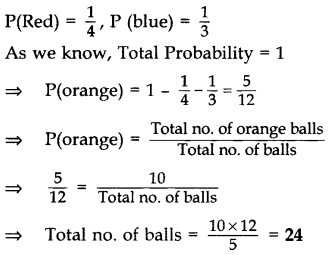Question 28.
A bag contains, white, black and red balls only. A ball is drawn at random from the bag. If the probability of getting a white ball is 3/10 and that of a black ball is 2/5, then find the probability of getting a red ball. If the bag contains 20 black balls, then find the total number of balls in the bag. (2015OD)
Solution:
Let W, B and R denote the White, Black and Red balls respectively,
We know, Total probability = 1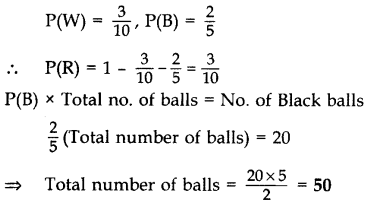Question 29.
A bag contains 15 white and some black balls. If the probability of drawing a black ball from the bag is thrice that of drawing a white ball, find the number of black balls in the bag. (2017OD)
Solution:
Let the number of black balls = x
the number of white balls = 15
∴ Total number of balls = x + 15
∴ P(black ball) = 3P(White balls)Question 30.
In a single throw of a pair of different dice, what is the probability of getting
(i) a prime number on each dice?
(ii) a total of 9 or 11? (2016D)
Solution:
Two dice can be thrown in 6 × 6 = 36 ways
(i) “a prime number on each dice” can be obtained as (2, 2), (2, 3), (2, 5), (3, 2), (3, 3), (3, 5), (5, 2), (5, 3), (5, 5), i.e., 9 ways.
∴ P(a prime no. on each dice) = 9/36=1/4

(ii) “a total of 9 or 11” can be obtained as (3, 6),(6, 3),(4, 5),(5, 4) (5, 6),(6, 5).
Total ‘9’ Total ’11’ i.e., 6 ways
∴ P(a total of 9 or 11) = 6/36=1/6

Question 31.
A box consists of 100 shirts of which 88 are good, 8 have minor defects and 4 have major defects. Ramesh, a shopkeeper will buy only those shirts which are good but ‘Kewal another shopkeeper will not buy shirts with major defects. A shirt is taken out of the box at random. What is the probability that:
(i) Ramesh will buy the selected shirt?
(ii) ‘Kewal will buy the selected shirt? (2016D)
Solution: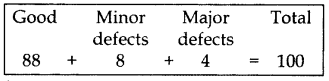No. of good shirts = 88
= P(good shirts) = 88/100=22/25

(ii) No. of shirts without major defect = 96
= P(shirts without major defect)
88+8/100=96/100=24/25

Question 32.
A card is drawn at random from a well shuffled pack of 52 cards. Find the probability of getting: (2012 OD)
(i) a red king.
(ii) a queen or a jack.
Solution:
Cards in a pack = 52
Number of kings = 4
Number of red kings = 2Question 33.
All red face cards are removed from a pack of playing cards. The remaining cards were well shuffled and then a card is drawn at random from them. Find the probability that the drawn card is (2015D)
(i) a red card
(ii) a face card
(iii) a card of clubs.
Solution:
Number of red face cards removed = 6
∴ Remaining cards = 52 – 6 = 46
Hence, Total no. of outcomes = 46
(i) Possible outcomes of red cards = 26 – 6 = 20
∴ P(a red card) = 20/46=10/23

(ii) Possible outcomes of face cards = 6
∴ P(a face card) = 6/46=3/23

(iii) Possible outcomes of card of clubs = 13
∴ P(a card of clubs) = 13/46

Question 34.
From a pack of 52 playing cards, Jacks, Queens and Kings of red colour are removed. From the remaining, a card is drawn at random. Find the probability that drawn card is:
(i) a black King
(ii) a card of red colour
(iii) a card of black colour (2016OD)
Solution:
Total cards in the pack = 52
Cards removed
= 2(Jacks) + 2(Queens) + 2(Kings) = 6
∴ Remaining cards = 52 – 6 = 46
(i) Number of black Kings = 2
∴ P(a black King) = 2/46=1/23

(ii) Total red cards in the pack = 26
Red cards removed = 6
Remaining red cards = 26 – 6 = 20
∴ P(a card of red colour) = 20/46=10/23

(iii) Total black cards in the pack = 26
∴ P(a card of black colour) = 26/46=13/23

Question 35.
There are 100 cards in a bag on which num bers from 1 to 100 are written. A card is taken out from the bag at random. Find the probability that the number on the selected card
(i) is divisible by 9 and is a perfect square
(ii) is a prime number greater than 80. (2016OD)
Solution:
Total cards = 100
(i) Numbers which are “Divisible by 9 and perfect squares” are 9, 36, 81, i.e., 3 .
∴ P(Divisible by 9 & perfect square) = 3/100

(ii) Numbers which are “prime numbers greater than 80” are 83, 89, 97, i.e., 3
∴ P(Prime nos. > 80) = 3/100

Question 36.
Two different dice are rolled together. Find the probability of getting: (2015 D)
(i) the sum of numbers on two dice to be 5.
(ii) even numbers on both dice.
Solution:
Total possible outcomes = 6n = 62 = 36
(i) The possible outcomes are (2, 3), (3, 2), (1, 4), (4, 1) when the sum of numbers on two dice is 5, i.e., 4
∴ Required Probability, P(E) = 4/36=1/9

(ii) The possible outcomes are (2, 2), (2, 4), (2, 6), (4, 2), (4, 4), (4, 6), (6, 2), (6, 4), (6, 6) for even numbers on both dice; 9
∴ Required Probability, P(E) = 9/36=1/4

Question 37.
Two different dice are thrown together. Find the probability of:
(i) getting a number greater than 3 on each die
(ii) getting a total of 6 or 7 of the numbers on two dice (2016D)
Solution:
Two dice can be thrown in 6 x 6 = 36 ways
(i) “getting a number > 3 on each die” can be obtained as (4, 4), (4, 5), (4, 6), (5, 4), (5, 5), (5, 6), (6, 4), (6, 5), (6, 6), i.e., 9 ways.
P(number > 3 on each die) = 9/36=1/4

(ii) “a total of 6 or 7 can be obtained as (2, 4),(4, 2),(3, 3), (1, 6),(6, 1),(2, 5) (1, 5),(5, 1) (5, 2),(3, 4),(4, 3), i.e., 11 ways
Total 6 Total “7′
P(a total of 6 or 7) = 11/36

Question 38.
Two different dice are thrown together. Find the probability that the numbers obtained.
(i) have a sum less than 6
(ii) have a product less than 16
(iii) is a doublet of odd numbers. (2017D)
Solution:
Two dice can be thrown in (6 × 6) = 36 ways(i) Sum less than ‘6’ are (1, 1) (1, 2) (1, 3) (1, 4), (2, 1) (2, 2) (2, 3) (3, 1) (3, 2) (4, 1) i.e., 10 ways
∴ P(sum < 6) = 10/36=5/18

(ii) Product less than ’16’ are (1, 1) (1, 2) (1, 3) (1, 4) (1, 5) (1, 6) (2, 1) (2, 2) (2, 3) (2, 4) (2,5) (2, 6) (3, 1) (3, 2) (3, 3) (3, 4) (3,5)(4, 1) (4, 2) (4, 3) (5, 1) (5, 2) (5,3) (6, 1) (6, 2) i.e., 25 ways
∴ P(product less than 16) = 25/36

(iii) (1, 1) (3, 3) (5, 5) i.e., 3 ways
∴ P(doublet of odd nos.) = 3/36=1/12

### Probability Class 10 Important Questions Long Answer (4 Marks)

Question 39.
A survey has been done on 100 people out of which 20 use bicycles, 50 use motorbikes and 30 use cars to travel from one place to another. Find the probability of persons who use bicycles, motorbikes and cars respectively? (2011D)
Solution: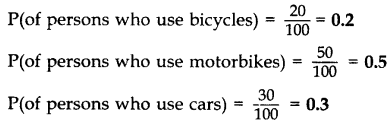Question 40.
A box contains 35 blue, 25 white and 40 red ‘marbles. If a marble is drawn at random from the box, find the probability that the drawn marble is (i) white (ii) not blue (iii) neither white nor blue. (2012D)
Solution:
No. of blue marbles = 35
No. of white marbles = 25
No. of red marbles = 40
Total no. of marbles = 35 + 25 + 40 = 100Question 41.
A box contains 70 cards numbered from 1 to 70. If one card is drawn at random from the box, find the probability that it bears
(i) a perfect square number.
(ii) a number divisible by 2 and 3. (2012OD)
Solution:
(i) Perfect squares upto 70 are
12, 22, …, 82 = 8
∴ P(a perfect square) = 8/70=4/35
(ii) Numbers divisible by 2 and 3 are:
6, 12, 18, …, 66, i.e., 11 nos.
∴ P(a no. divisible by 2 and 3) = 11/70

Question 42.
A group consists of 12 persons, of which 3 are extremely patient, other 6 are extremely honest and rest are extremely kind. A person from the group is selected at random. Assuming that each person is equally likely to be selected, find the probability of selecting a person who is (2013D)
(i) extremely patient
(ii) extremely kind or honest.
Solution:
Extremely patient = 3
Extremely honest = 6
Extremely kind = 12 – 3 – 6 = 3Question 43.
A box contains cards numbered 3, 5, 7, 9, …, 35, 37. A card is drawn at random from the box. Find the probability that the number on the drawn card is a prime number. (2013OD)
Solution:
Total number of cards = 18
Prime numbers are: 3, 5, 7, 11, 13, 17, 19, 23, 29, 31, 37, i.e., 11
∴ P(Prime number) = 11/180

Question 44.
Find the probability that a leap year selected at random, will contain 53 Mondays. (2013OD)
Solution:
In a leap year, total number of days = 366
∴ 366 days
= 52 complete weeks + 2 extra days
Thus, a leap year always has 52 Mondays and extra 2 days.
Extra 2 days can be,
(i) Sunday and Monday
(ii) Monday and Tuesday
(iii) Tuesday and Wednesday
(iv) Wednesday and Thursday
(v) Thursday and Friday
(vi) Friday and Saturday
(vii) Saturday and Sunday
Let E be the event that a leap year has 53 Mondays.
∴ E = {Sun and Mon, Mon and Tues}
∴ P(E) = 2/7

Question 45.
A bag contains cards numbered from 1 to 49. A card is drawn from the bag at random, after mixing the cards thoroughly. Find the probability that the number on the drawn card is:
(i) an odd number
(ii) a multiple of 5
(iii) a perfect square
(iv) an even prime number (2014D)
Solution:
Total number of cards = 49
(i) Odd numbers are 1, 3, 5, …., 49, i.e., 25
∴ P(an odd number) = 25/49

(ii) ‘A multiple of 5’ numbers are 5, 10, 15, ……., 45, i.e., 9
∴ P(a multiple of 5) = 9/49

(iii) “A perfect square” numbers are 1, 4, 9, …….., 49, i.e., 7
∴ P(a perfect square number) = 7/49=1/7

(iv) “An even prime number” is 2, i.e., only one number
∴ P(an even prime number) = 1/49

Question 46.
A box contains 20 cards numbered from 1 to 20. A card is drawn at random from the box. Find the probability that the number on the drawn card is (2015D)
(i) divisible by 2 or 3,
(ii) a prime number.
Solution:
(i) Numbers divisible by 2 or 3 from 1 to 20 are 2, 4, 6, 8, 10, 12, 14, 16, 18, 3, 9, 15, 20 = 13
Total outcomes = 20
Possible outcomes = 13
∴ P(divisible by 2 or 3) = 13/20

(ii) Prime numbers from 1 to 20 are 2, 3, 5, 7, 11, 13, 17, 19 = 8
Total Outcomes = 20
Possible outcomes = 8
∴ P(a prime number) = 8/20=2/5

Question 47.
A bag contains 25 cards numbered from 1 to 25. A card is drawn at random from the bag. Find the probability that the number on the drawn card is: (2015D)
(i) divisible by 3 or 5
(ii) a perfect square number.
Solution:
Total number of outcomes = 25
(i) Possible outcomes of numbers divisible by 3 or 5 in numbers 1 to 25 are (3, 6, 9, 12, 15, 18, 21, 24, 5, 10, 20, 25) = 12
∴P(No. divisible by 3 or 5) = 12/25

(ii) Possible outcomes of numbers which are a perfect square = 5, i.e., (1, 4, 9, 16, 25)
∴ P(a perfect square no.) = 5/25=1/5

Question 48.
A game of chance consists of spinning an arrow on a 3 circular board, divided into / 4 8 equal parts, which comes to rest pointing at one of the numbers 1, 2, 3, …, 8 (Figure), which are equally likely outcomes. What is the probability that the arrow will point at
(i) an odd number
(ii) a number greater than 3
(iii) a number less than 9. (2016 D)Solution:
Total numbers = 8
(i) “Odd numbers” are 1, 3, 5, 7, i.e., 4
∴ P(an odd number) = 4/8=1/2

(ii) “nos. greater than 3” are 4, 5, 6, 7, 8, i.e., 5
∴ P(a number > 3) = 5/8

(iii) “numbers less than 9” are 1, 2, 3, …8 i.e., 8
∴ P(a number < 9) = 8/8 = 1

Question 49.
A number x is selected at random from the numbers 1, 2, 3 and 4. Another number y is selected at random from the numbers 1, 4, 9 and 16. Find the probability that product of x and y is less than 16. (2016OD)
Solution:
X can be any one of 1, 2, 3, and 4 i.e., 4 ways
Y can be any one of 1, 4, 9, and 16 i.e., 4 ways
Total no. of cases of XY = 4 × 4 = 16 ways
No. of cases, where product is less than 16 (1, 1), (1, 4), (1, 9), (2, 1), (2, 4), (3, 1), (3, 4), (4,1) i.e., 8 ways
∴ P (product x & y less then 16) = 8/16=1/2

Question 50.
The probability of guessing the correct answer to a certain question is x/12 . If the probability of guessing the wrong answer is 3/4 , find x. If a student copies the answer, then its probability is 2/6 . If he doesn’t copy the answer, then the probability is 2y/3 . Find the value of y. (2011OD)
Solution:
P (guessing) + P (guessing wrong) = 1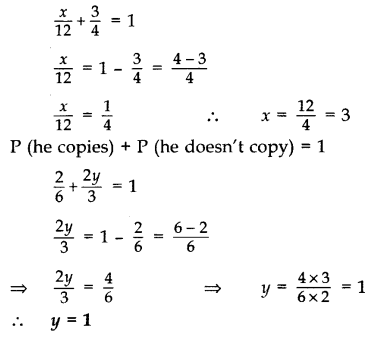Question 51.
A number x is selected at random from the numbers 1, 4, 9, 16 and another number y is selected at random from the numbers 1, 2, 3, 4. Find the probability that the value of xy is more than 16. (2016OD)
Solution:
x can be any one of 1, 4, 9, or 16, i.e., 4 ways
y can be any one of 1, 2, 3, or 4, i.e., 4 ways
Total number of cases of xy = 4 × 4 = 16 ways
Number of cases, where product is more than 16
(9, 2), (9, 3), (9, 4), (16, 2), (16, 3), (16, 4), i.e., 6 ways
∴ Required probability = 6/16=3/8

Question 52.
A game consists of tossing a coin 3 times and noting its outcome each time. Hanif wins if he gets three heads or three tails, and loses otherwise. Calculate the probability that Hanif will lose the game. (2011D)
Solution:
The possible outcomes on tossing a coin 3 times are,
S = {HHH, HHT, HTH, THH, TTH, THT, HTT, TTT) = 8
Outcomes when Hanif wins = {HHH, TTT} = 2
∴ P (Hanif wins) = 2/8=1/4
∴ P (Hanif will lose) = 1 – 1/4=3/4

Question 53.
Two dice are rolled once. Find the probability of getting such numbers on the two dice, whose product is 12. (2011OD)
Solution:
Two dice can be thrown in 6 × 6 = 36 ways
‘Product is 12′ can be obtained as (2, 6), (6, 2), (3, 4), (4, 3), i.e., in 4 ways
∴ P(Product is 12) = 4/36=1/9

Question 54.
A box contains 100 red cards, 200 yellow cards and 50 blue cards. If a card is drawn at random from the box, then find the probability that it will be
(i) a blue card
(ii) not a yellow card
(iii) neither yellow nor a blue card. (2012D)
Solution:
No. of red cards = 100
No. of yellow cards = 200
No. of blue cards = 50
Total no. of cards = 100 + 200 + 50 = 350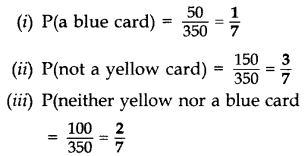Question 55.
Two different dice are thrown together. Find the probability that the numbers obtained have (2017OD)
(i) even sum, and
(ii) even product.
Solution:
Two dice can be thrown as 6 × 6 = 36 ways.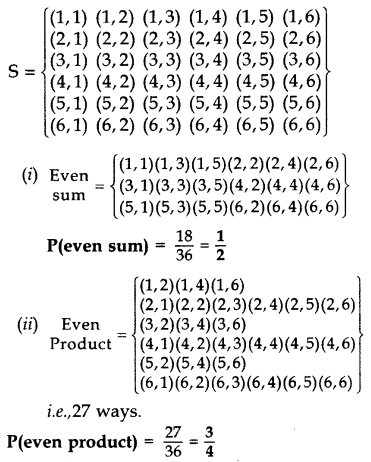Question 56.
A card is drawn from a well shuffled deck of 52 cards. Find the probability of getting
(i) a king of red colour
(ii) a face card
(iii) the queen of diamonds. (2012 D)
Solution:
(i) Total cards in a deck = 52
Total no. of kings = 4
Total no. of red kings = 2Question 57.
All kings, queens and aces are removed from a pack of 52 cards. The remaining cards are well shuffled and then a card is drawn from it. Find the probability that the drawn card is
(i) a black face card.
(ii) a red card. (2012OD)
Solution:
Total no. of cards = 52
No. of cards removed = (4 + 4 + 4) = 12
Remaining cards = 40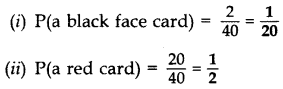Question 58.
Red kings and black aces are removed from a pack of 52 cards. The remaining cards are well shuffled and then a card is drawn from it. Find the probability that the drawn card is
(i) a black face card.
(ii) a red card. (2012OD)
Solution:
Total nos. of cards = 52
Cards removed = 2 + 2 = 4
Remaining cards = 52 – 4 = 48Question 59.
All the black face cards are removed from a pack of 52 playing cards. The remaining cards are well shuffled and then a card is drawn at random. Find the probability of getting a:
(i) face card
(ii) red card
(iii) black card
(iv) king (2014D)
Solution:
Total number of cards = 52
Black face cards = 6
Remaining cards = 52 – 6 = 46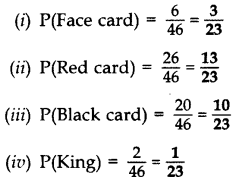Question 60.
Cards numbered from 11 to 60 are kept in a box. If a card is drawn at random from the box, find the probability that the number on the drawn card is: (2014D)
(i) an odd number
(ii) a perfect square number
(iii) divisible by 5
(iv) a prime number less than 20
Solution:
Total number of cards = 60 – 11 + 1 = 50
(i) Odd nos, are 11, 13, 15, 17, …. 59 = 25 no.
∴ P(an odd number) = 25/50=1/2

(ii) Perfect square numbers are 16, 25, 36, 49 = 4 numbers
∴ P(a perfect square no.) = 4/50=2/25

(iii) “Divisible by 5” numbers are 15, 20, 25, 30, 35, 40, 45, 50, 55, 60, = 10 numbers
∴ P(divisible by 5) = 10/50=2/25

(iv) Prime numbers less than 20 are 2, 3, 5, 7, 11, 13, 17, 19 = 8 numbers
∴ P(a prime no. less than 20) = 8/50=4/25

Question 61.
Red queens and black jacks are removed from a pack of 52 playing cards. A card is drawn at random from the remaining cards, after reshuffling them. Find the probability that the drawn card is (2014OD)
(i) a king
(ii) of red colour
(iii) a face card
(iv) a queen
Solution:
Number of red queens = 2
Number of black jacks = 2
Remaining cards = 52 – 2 – 2 = 48Question 62.
All the red face cards are removed from a pack of 52 playing cards. A card is drawn at random from the remaining cards, after reshuffling them. Find the probability that the drawn card is (2014OD)
(i) of red colour
(ii) a queen
(iii) an ace
(iv) a face card
Solution:
Total number of cards = 52
Red face cards = 6
Remaining cards = 52 – 6 = 46Question 63.
Five cards, the ten, jack, queen, king and ace of diamonds, are well shuffled with their faces downwards. One card is then picked up at random. (2014OD)
(a) What is the probability that the drawn card is the queen?
(b) If the queen is drawn and put aside, and a second card is drawn, find the probability that the second card is (i) an ace (ii) a queen.
Solution:
(a) Total events = 5; P(queen) = 1y/5
(b) Now total events = 4
(i) P (an ace) = 1/4
(ii) P (a queen) = 0/4 = 0 …[As there is no queen left

Question 64.
A card is drawn at random from a well shuffled deck of playing cards. Find the probability that the card drawn is (2015OD)
(i) a card of spade or an ace.
(ii) a black king.
(iii) neither a jack nor a king.
(iv) either a king or a queen.
Solution:
Total no. of outcomes = 52## CBSE Class 10 Maths Notes Chapter 15 Probability

Probability: It is the numerical measurement of the degree of certainty.

• Theoretical probability associated with an event E is defined as “If there are ‘n’ elementary events associated with a random experiment and m of these are favourable to the event E then the probability of occurrence of an event is defined by P(E) as the ratio m/n “.• If P(E) = 1, then it is called a ‘Certain Event’.
• If P(E) = 0, then it is called an ‘Impossible Event’.
• The probability of an event E is a number P(E) such that: 0 ≤ P(E) ≤ 1
• An event having only one outcome is called an elementary event. The sum of the probabilities of all the elementary events of an experiment is 1.
• For any event E, P(E) + P(E¯) = 1, where E¯ stands for ‘not E’. E and E¯ are called complementary events.
• Favourable outcomes are those outcomes in the sample space that are favourable to the occurrence of an event.

Sample Space
A collection of all possible outcomes of an experiment is known as sample space. It is denoted by ‘S’ and represented in curly brackets.
Examples of Sample Spaces:
A coin is tossed = Event
E1 = Getting a head (H) on upper face
E2 = Getting a tail (T) on upper face
S = {H, T}
Total number of outcomes = 2

Two coins are tossed = Event = E
E1 = Getting a head on coin 1 and a tail on coin 2 = (H, T)
E2 = Getting a head on both coin 1 and coin 2 = (H, H)
E3 = Getting a tail on coin 1 and a head on coin 2 = (T, H)
E4 = Getting a tail on both, coin 1 and coin 2 = (T, T)
S = {(H, T), (H, H), (T, H), (T, T)}.
Total number of outcomes = 4

NOTE: In probability the order in which events occur is important
E1 & E3 are treated as different outcomes.

Important Tips

• Coin: A coin has two faces termed as Head and Tail.
• Dice: A dice is a small cube which has between one to six spots or numbers on its sides, which is used in games.
• Cards: A pack of playing cards consists of four suits called Hearts, Spades, Diamonds and Clubs. Each suite consists of 13 cards.

## SabDekho

The Complete Educational Website